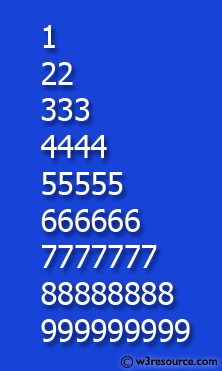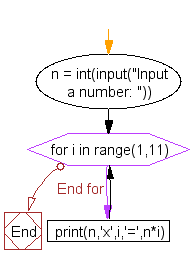﻿ Python Exercise: Construct the following pattern, using a nested loop number - w3resource# Python Exercise: Construct the following pattern, using a nested loop number

## Python Conditional: Exercise - 44 with Solution

Write a Python program to construct the following pattern, using a nested loop number.

Pictorial Presentation:Sample Solution:

Python Code:

``````for i in range(10):
print(str(i) * i)
```
```

Sample Output:

```1
22
333
4444
55555
666666
7777777
88888888
999999999
```

Flowchart :## Visualize Python code execution:

The following tool visualize what the computer is doing step-by-step as it executes the said program:

Python Code Editor:

Have another way to solve this solution? Contribute your code (and comments) through Disqus.

What is the difficulty level of this exercise?

Test your Python skills with w3resource's quiz

﻿

## Python: Tips of the Day

Returns a list with n elements removed from the beginning

Example:

```def tips_take(itr, n = 1):
return itr[:n]
print(tips_take([1, 2, 3], 5))
print(tips_take([1, 2, 3], 0))
```

Output:

```[1, 2, 3]
[]
```